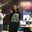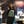Trusted answers to developer questions
Trusted Answers to Developer Questions

Related Tags

# What is the epsilon NFA?Ayesha Kanwal

Grokking Modern System Design Interview for Engineers & Managers

Ace your System Design Interview and take your career to the next level. Learn to handle the design of applications like Netflix, Quora, Facebook, Uber, and many more in a 45-min interview. Learn the RESHADED framework for architecting web-scale applications by determining requirements, constraints, and assumptions before diving into a step-by-step design process.

### Overview

An epsilon nondeterministic finite automaton (NFA) has null or epsilon transitions from one state to another. Epsilon NFA is also called a null NFA or an NFA lambda.

A regular expression for a language forms an epsilon NFA. This epsilon NFA then converts to a simple NFA. We then use the simple NFA to make a deterministic finite automaton (DFA).

### Null closure

We require null closure to convert an epsilon NFA to an NFA. Moreover, it shows us the states we can transcend due to a null transition.

Note: We can visit here to learn how to convert an epsilon NFA to NFA.

Let's suppose an NFA $$ and $S \subseteq Q$ is a defined set of states, where:

• $Q$ is the finite set of states
• $Σ$ is the input symbols
• $q_0$ is the start state
• $δ$ is the transition function
• $F$ is the final state

The null closure of $S$ is the set $Λ(S)$. We can define it recursively as follows:

1. $S \subseteq Λ(S)$
2. For every $q \in Λ(S)$, $δ(q, Λ) \subseteq Λ(S)$

### Example

Consider the following epsilon NFA, where $q_0$ is the start state.

An epsilon NFA

The null closure of the NFA is as follows:

Null closure
 State Set q0 {q0, q1, q3} q1 {q1, q3} q2 {q2} q3 {q3}

We determine this null closure by how a transition transcends from one state to another with a null input.

RELATED TAGS

CONTRIBUTORAyesha Kanwal